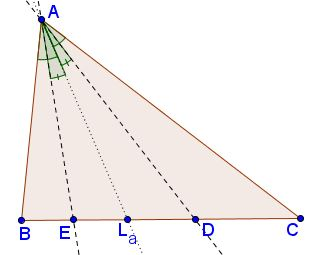# Steiner's Ratio Theorem

Let $D\;$ be a point on the sideline $BC\;$ of $\Delta ABC,\;$ and the reflection of the line $AD\;$ in the internal angle bisector of the angle $A\;$ intersect the line $BC\;$ at a point $E.\;$Then

$\displaystyle\frac{BD}{CD}\cdot\frac{BE}{CE}=\frac{AB^2}{AC^2}.$

Lines $AD\;$ and $AE\;$ are said to be isogonal (or isogonal conjugate).

### Proof

By the Law of Sines,

$\displaystyle\frac{BD}{AB}=\frac{\sin\angle BAD}{\sin\angle ADB}$ and $\displaystyle\frac{CD}{AC}=\frac{\sin\angle CAD}{\sin\angle ADC}=\frac{\sin\angle CAD}{\sin\angle ADB}.$

Dividing the two term-wise gives $\displaystyle\frac{BD}{CD}=\frac{AB}{AC}\frac{\sin\angle BAD}{\sin\angle CAD}.$

Similarly, $\displaystyle\frac{BE}{CE}=\frac{AB}{AC}\frac{\sin\angle BAE}{\sin\angle EAC}.$

The product of the two reduces to the required $\displaystyle\frac{BD}{CD}\cdot\frac{BE}{CE}=\frac{AB^2}{AC^2}$ because, by the construction, $\angle BAD=\angle EAC\;$ and $\angle BAE =\angle CAD.\;$

### Corollary

Point $X\;$ on the side $BC\;$ of $\Delta ABC\;$ has the property that $\displaystyle\frac{BX}{CX}=\frac{AB^2}{AC^2}\;$ iff $AX\;$ is the symmedian through vertex $A.$

### References

1. Sammy Luo and Cosmin Pohoata, Let's Talk About Symmedians!, Mathematical Reflections 4 (2013), 1-11### Symmedian